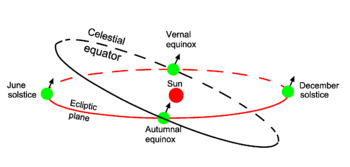# Ecliptic

In astronomy, the ecliptic is the great circle on the celestial sphere that the Sun, seen from the Earth, seems to describe in the sky. The ecliptic lies in the ecliptic plane that by definition contains the Earth and the apparent orbit of the Sun; see Fig. 1. Equivalently, the ecliptic plane may be defined as the plane of the Sun and the orbit of the Earth; see Fig. 2.Fig. 2. The Earth in its orbit around the Sun. The arrow on the Earth indicates the angle between the Earth axis and the ecliptic plane; it also gives the direction of the diurnal (daily) rotation of the Earth by the right-hand rule.

The Earth is the center of the celestial sphere and the celestial equator is a circle in the same plane as the equator of the Earth; the two equators are coplanar and concentric. The plane of the two equators (of the Earth and of the celestial sphere) makes an angle of approximately 23.43° = 23° 26' with the ecliptic plane; this angle is known as the obliquity of the ecliptic. In Fig. 2 one sees that the angle is the complement of the angle (66.57°) that the rotation axis (North-South axis) of the Earth makes with the ecliptic plane. The angle 66.57° is the latitude of the polar circles. (More precisely the latitude was in 2010: 66˚ 33' 43", because of a small precession of the Earth's axis the number shows small variations in time).

The intersections of the celestial equator and the ecliptic are the vernal equinox (the date in the spring of the Northern hemisphere that day and night are equally long, around March 21) and the autumnal equinox (the date in the fall that day and night are equally long, around September 21). The solstices are the dates that the Sun seems to stand still (around June 21 and December 21).